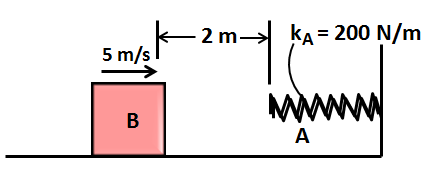# The 7.6 ''kg'' block is moving with an initial speed of 5''m''/''s''. The coefficient of kinetic...

## Question:

The 7.6 kg block is moving with an initial speed of 5m/s. The coefficient of kinetic friction between the block and plane is {eq}\mu _{k}=0.25 {/eq}.

Determine the compressions in the spring when the block momentarily stops.## Kinetic Energy

When a body is in motion there is an energy associated with it. This is the kinetic energy of the body. Kinetic energy is proportional to the square of the velocity of the body. So when the velocity of the body increases, the kinetic energy increases by the square of the increase in velocity.

As the block moves towards the spring it loses kinetic energy due to frictional losses and also due to spring force acting against it. This continues until the body comes to a rest.

Let the compression in the spring be x when the block comes to a rest.

The energy balance equation would be,

Kinetic energy - Frictional work - Work done by spring = 0

{eq}\displaystyle \frac {1}{2}mv^2 - \mu_k mg (2 +x) - \frac{1}{2}k_A x^2 = 0 \\ \displaystyle \frac{1}{2}*7.6*5^2 - 0.25*7.6*9.81*(2+x) - \frac{1}{2}*200*x^2 = 0 \\ 95 - 18.639(2+x) - 100x^2 = 0 \\ 100x^2 + 18.639x - 57.722 = 0 \\ x = 0.672 \ m {/eq}

This is the compression of the spring when the body comes to a rest temporarily.